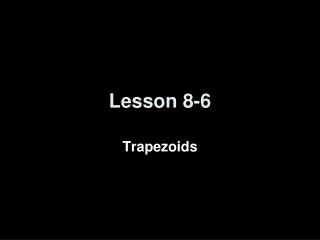DownloadDownload PresentationLesson 8-6

# Lesson 8-6

Télécharger la présentation## Lesson 8-6

- - - - - - - - - - - - - - - - - - - - - - - - - - - E N D - - - - - - - - - - - - - - - - - - - - - - - - - - -
##### Presentation Transcript

1. Lesson 8-6 Trapezoids

2. Transparency 8-6 5-Minute Check on Lesson 8-5 L • LMNO is a rhombus. • Find x • Find y • QRST is a square. • Find n if mTQR = 8n + 8. • Find w if QR = 5w + 4 and RS = 2(4w – 7). • Find QU if QS = 16t – 14 and QU = 6t + 11. • 6. What property applies to a square, but not to a rhombus? (8y – 6)° (3x + 12)° 7 M O P 12 (5x – 2)° N Q R 10.25 U 6 T S 65 Standardized Test Practice: A C Opposite sides are congruent Diagonals bisect each other D B D Opposite angles are congruent All angles are right angles Click the mouse button or press the Space Bar to display the answers.

3. Objectives • Recognize and apply the properties of trapezoids • Solve problems involving medians of trapezoids

4. Vocabulary • Trapezoid – a quadrilateral with only one pair of parallel sides • Isosceles Trapezoid – a trapezoid with both legs (non parallel sides) congruent • Median – a segment that joins the midpoints of the legs of a trapezoid

5. Polygon Hierarchy Polygons Quadrilaterals Parallelograms Kites Trapezoids IsoscelesTrapezoids Rectangles Rhombi Squares

6. Trapezoids Trapezoid CharacteristicsBases Parallel Legs are not Parallel Leg angles are supplementary (mA + mC = 180, mB + mD = 180) Median is parallel to basesMedian = ½ (base + base)½(AB + CD) base A B legmidpoint legmidpoint median C D base A B Isosceles Trapezoid CharacteristicsLegs are congruent (AC  BD) Base angle pairs congruent (A  B, C  D) Diagonals are congruent (AD  BC) M C D

7. DEFG is an isosceles trapezoid with median Find DG if and Answer: EXAMPLE 1 Example 6-4a Theorem 8.20 Substitution Multiply each side by 2. Subtract 20 from each side.

8. DEFG is an isosceles trapezoid with median Find , and if and EXAMPLE 1 (CONT) Example 6-4c Since EF // DG, 1 and 3 are supplementary Because this is an isosceles trapezoid, 1  2 and 3  4 Substitution Combine like terms. Divide each side by 9. Answer: If x = 20, then m1 = 65° and 3 = 115°. Because 1  2 and 3  4, 2 = 65° and 4 = 115°

9. WXYZ is an isosceles trapezoid with median a. Answer: b. Answer: Because EXAMPLE 2 Example 6-4e

10. Quadrilateral Characteristics Summary Convex Quadrilaterals 4 sided polygon 4 interior angles sum to 360 4 exterior angles sum to 360 Parallelograms Trapezoids Bases Parallel Legs are not Parallel Leg angles are supplementary Median is parallel to basesMedian = ½ (base + base) Opposite sides parallel and congruent Opposite angles congruent Consecutive angles supplementary Diagonals bisect each other Rectangles Rhombi IsoscelesTrapezoids All sides congruent Diagonals perpendicular Diagonals bisect opposite angles Angles all 90° Diagonals congruent Legs are congruent Base angle pairs congruent Diagonals are congruent Squares Diagonals divide into 4 congruent triangles

11. Summary & Homework • Summary: • In an isosceles trapezoid, both pairs of base angles are congruent and the diagonals are congruent. • The median of a trapezoid is parallel to the bases and its measure is one-half the sum of the measures of the bases • Homework: • Pg 442 (13-18) • Pg 445 (practice quiz… 1, 2, 4, 5)